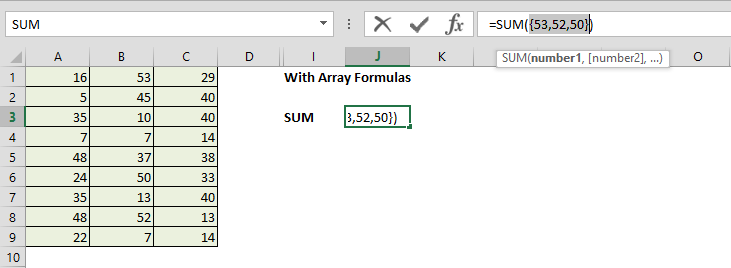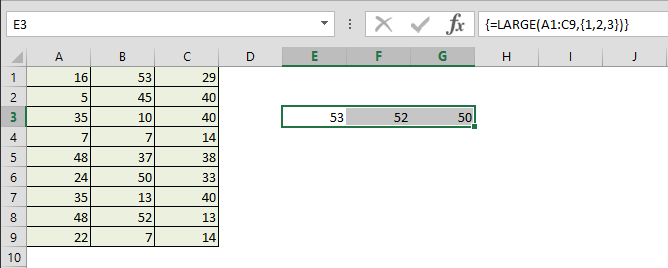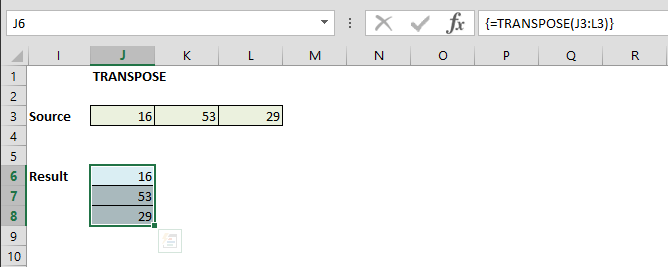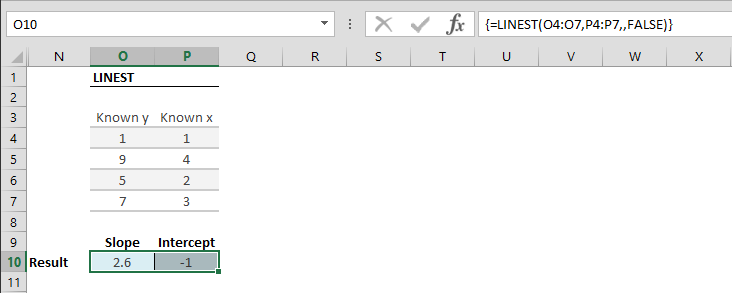Excel’s array formulas are indeed a fascinating subject. Although they can be very useful by making it possible to solve some complex calculations, and replace hundreds of formulas in certain situations, most Excel users have probably never used these formulas before and didn’t even feel a difference. Let us show you how array formulas can change the way you create your Excel models.

The word array in Excel refers to an arrangement of items. It’s essentially a table of values that Excel can keep in its memory for use in other operations. Array items can come from any number of cells or user inputs.

If you’re trying to do any of the following, array formulas are the way to go,

• Sum every nth value or every other value in a range
• Count the number of all or some characters in a range
• Sum numbers that meet certain conditions (i.e. the lowest/highest values in a range that fall between specific boundaries)

Array formulas can return results into either a single cell or multiple cells. We’re going to get more into this later when covering multi-cell arrays.

Let’s take an example. Assume that we need to find sum of n number of largest values from a table. Without an array formula, we can just calculate the largest values (there’s going to be n of them) and then add them up separately. You can download the workbook we’re going to be using from this link.

In the example below we have a set of numbers in A1:C9 and the sum of the 3 largest numbers is what we’re looking for. First step is the largest values using the LARGE function. Entering the same formula for all 3 rows, we get the first, second, and third largest numbers in the table.We can now use a SUM formula to add these numbers to get the result below.Now, let’s take a look at how the array formula would’ve worked in this scenario. We can tell Excel to use our table to run the calculations. Every operation we did before can be handled in a single cell with this special formula and this is going to help us save time and space. Here, we can nest the two formulas and press Ctrl + Shift + Enter to make it an array formula.Doing this will add curly braces to the formula. Don’t worry, you don’t need to add them when doing this. This is simply how Excel shows that this is now an array formula. Our formula tells Excel to use the LARGE function calculated for all values (1, 2, and 3 in this case) and return an array of results into SUM function.After selecting the formula, you can use Excel’s Evaluate Formula feature or press F9 to see the calculated totals.## Multi-Cell Array Formulas

We mentioned that array formulas can return results in a single cell or multiple cells. Taking it one step further, we can also use array formulas to print the outcome into separate cells. Now, let’s take a look at a multi-cell example.

In the previous example, we used Excel’s LARGE function with an array of arguments to return the array into a SUM function. Let’s see what we could do if we wanted to print those values instead of using them in another function.

Begin by selecting a print range. Here, we selected a range covering one row and three columns (E through G).Next, enter your formula into the formula bar and press Ctrl + Shift + Enter to enter it as an array formula.The results will now be displayed in the selected range.Array formulas work a bit differently than regular Excel functions. One of the biggest differences is that you can’t edit or clear any one of the cells individually. You must select all three to manipulate this range.

Another side note here is that your range selection must match the output layout of the formula you are using. In our example, the LARGE formula gives a set of three values and therefore we selected a 1x3 grid. In other words, you must use a single row and multiple columns for single dimensional arrays and multiple rows and columns if your formula returns a two dimensional array. If an array returned by the formula is smaller than the selected range, Excel will give #N/A errors in the overflow cells.

## Common Formulas

Let’s take a look at some of the typical array functions and functions that return an array as a result. While some of these formulas can only be used as an array formula, like the TRANSPOSE function, some will return a value that will be recognized as the first element of an array like LINEST function. Common examples are,

• TRANSPOSE
• INDEX
• OFFSET
• LINEST
• LOGEST

#### TRANSPOSE

TRANSPOSE function copies the contents of a range into another range by converting rows into columns and vice-versa. If you use the TRANSPOSE function without pressing Ctrl + Shift + Enter, the first value of your range selection will give a #VALUE! error.#### LINEST

The LINEST function calculates a best fit line that matches the trend of your data by using the "least squares" method. This will essentially return slope and intercept values of the best fit line. Because the function returns more than one number, it is more suitable to use it as an array formula. To get the two values slope and intercept, select the two horizontal cells and then enter formula pressing Ctrl + Shift + Enter.When not used as an array function, LINEST will only return the slope value.

Array formulas add another dimension to what you can do with the endless world of Excel. They can be a bit tricky to use, but once you get a hang of it, you’re going to be surprised how you never heard of this powerful feature.